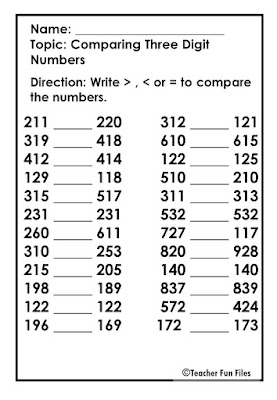Comparing Numbers Worksheet

Free Math worksheets about comparing numbers.
Direction: Write > greater than, < less than or = equal sign to compare the numbers.Tags: Math, comparing numbers, greater than, less than, equal, free worksheet, mathematics worksheet, comparing one digit numbers, comparing two digit numbers, comparing three digit numbers.